Next: FFTRN: Multi-Dimensional Real Fourier Up: How FFTEASY works Previous: FFTCN: Multi-Dimensional Complex Fourier

## FFTR1: One-Dimensional Real Fourier Transforms

The trick to doing a DFT of real data is to once again use the DL formula. Specifically the routine begins by taking the original array and passing it as is to fftc1. Of course fftc1 treats the data as an array of complex numbers. Note, however, that the real parts of those numbers are the elements of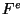and the imaginary parts are the elements of, so it is possible to extract the actual DFT of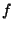from the results of fftc1. Let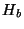represent the output of fftc1 and letrepresent the desired output, i.e. the transform of the real function. The input we gave to fftc1 was the combination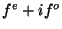so by the linearity of Fourier transforms we know that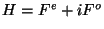. From the DL formula we also know that. How can we extractandfromin order to plug them into the DL formula? The answer lies in the symmetry relation obeyed by the Fourier transforms of real data (which includesand),. So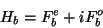(11)(12)

and thus(13)(14)

Plugging these equations into the DL formula gives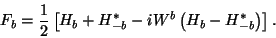(15)

Now all that's left is to figure out where all of these terms are stored in the array. Remember that all the array indices in these formulas are complex. In the case offinding the elements is trivial because only non-negative values of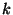are given as output so the elementsare stored in order. (The case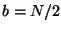is treated separately below.) Recall, however, thatis the transform of a complex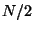size array, so its elements range fromtoin the wrap-around storage order described in section 2.3.2. In other words the value of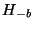is stored in the location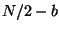. So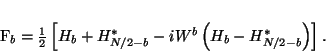(16)

Plugging infor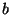in this equation and noting that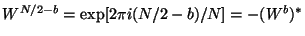gives(17)

Using these two equations the program steps over all the elements of the array overwritingand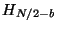withand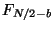. The casesandare a little different, however, becauseandare both real. The function fftr1 placesin the first (real) slot of theelement of the array andin the second (imaginary) slot. From the formulas above, noting that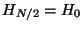by periodicity, we see that(18)(19)

Inverting this process seems like a mess but actually works out to be nearly identical. I won't reproduce all the algebra here but if you invert the formulas above to findfromyou will find that except for a couple of minus signs and a factor of two for the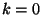andterms the results look identical to the forward case. As a result the inverse transform simply uses the same loop to rearrange the array and then calls fftc1 to do an inverse transform and get the function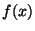back.Next: FFTRN: Multi-Dimensional Real Fourier Up: How FFTEASY works Previous: FFTCN: Multi-Dimensional Complex Fourier